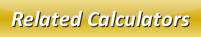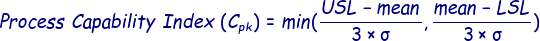# Cpk Calculator

In statistics, process capability index (Cpk) is very helpful for measuring the capability of a specific process to deliver its outcome as per the overall specification.

The process capability index is calculated with the help of this below formula:In the below online process capability index calculator, enter the upper specification limit, lower specification limit, standard deviation and mean in the respective input boxes and then click calculate to find out the output.

 Upper Specification Limit (USL): Lower Specification Limit (LSL): Standard Deviation (σ): Mean (μ): Process Capability Index (Cpk):

Latest Calculator Release

Average Acceleration Calculator

Average acceleration is the object's change in speed for a specific given time period. ...

Free Fall Calculator

When an object falls into the ground due to planet's own gravitational force is known a...

Torque Calculator

Torque is nothing but a rotational force. In other words, the amount of force applied t...

Average Force Calculator

Average force can be explained as the amount of force exerted by the body moving at giv...

Angular Displacement Calculator

Angular displacement is the angle at which an object moves on a circular path. It is de...